# MCAT Physical : Vapor Pressure

## Example Questions

2 Next →

### Example Question #11 : Vapor Pressure

Colligative properties are properties of compounds that are altered by the amount of substance present. There are four main colligative properties: boiling point, freezing point, vapor pressure, and osmotic pressure. The change in each of these properties can be calculated using the amount of molecules/ions present in solution and the concentration or partial pressure of the compound. The boiling point is defined as the temperature at which the vapor pressure equals the atmospheric pressure. The freezing point is the temperature at which a liquid is converted to a solid. Vapor pressure is the pressure produced by the vapor above a solution. Osmotic pressure is the pressure required to prevent flow of water into a solution (across a membrane).

Upon addition of salt, a researcher notices that there is an increase in the boiling point of the solution. Which of the following could be the reason for this observation?

The amount of particles escaping the solution is higher

The enthalpy of the solution increases

The pressure above the solution is higher than the atmospheric pressure

The pressure above the solution is lower than the atmospheric pressure

The pressure above the solution is lower than the atmospheric pressure

Explanation:

The boiling point is defined as the temperature at which the vapor pressure above the solution equals the atmospheric pressure. Recall that vapor pressure is also a colligative property. The vapor pressure decreases as solutes are added. This means that more energy, in the form of heat, is required to increase the amount of molecules escaping the solution and, subsequently, increase the vapor pressure to that of the atmospheric pressure. This increased demand of energy results in an increased boiling point.

### Example Question #12 : Vapor Pressure

Colligative properties are properties of compounds that are altered by the amount of substance present. There are four main colligative properties: boiling point, freezing point, vapor pressure, and osmotic pressure. The change in each of these properties can be calculated using the amount of molecules/ions present in solution and the concentration or partial pressure of the compound. The boiling point is defined as the temperature at which the vapor pressure equals the atmospheric pressure. The freezing point is the temperature at which a liquid is converted to a solid. Vapor pressure is the pressure produced by the vapor above a solution. Osmotic pressure is the pressure required to prevent flow of water into a solution (across a membrane).

The vapor pressure above a solvent is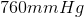. What is the change in vapor pressure if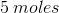of glucose is added to the solvent? Assume that there areof water present.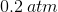Cannot be determined from the given information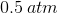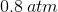Cannot be determined from the given information

Explanation:

Vapor pressure change is calculated using the following equation.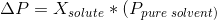where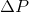is change in vapor pressure,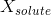is mole fraction of solute, and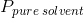is vapor pressure above pure solvent. First, let’s calculate the mole fraction.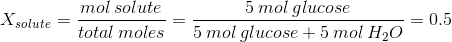The vapor pressure of pure solvent is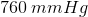. Recall that there arein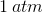; therefore, the vapor pressure of pure solvent is. We can now solve for the change in vapor pressure.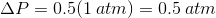This means that the vapor pressure decreased byafter the addition of glucose.

Note that vapor pressure also depends on the amount of ions.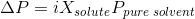wherethe number of ions. Glucose does not dissolve into ions; therefore,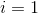. If we were given another molecule, such as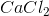, then we will have to set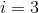and calculateaccordingly.

2 Next →

### All MCAT Physical Resources